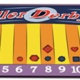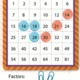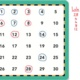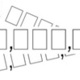Display Accessibility Tools

# Math Games

Have fun while learning or reviewing mathematics at home. These games are offer math learning opportunities while challenging students in a game setting. These can be used in all 3 grade levelsStudents are developing basic knowledge of probability and how knowledge of probability helps in making better decisions. Analyzing the game can also reinforce their understanding of fractions, ratios, and proportional reasoning.Students are developing and reinforcing their knowledge of multiplication and division as inverse operations, factors, and products. Analyzing the game can also reinforce their understanding of basic number theory including prime and composite numbers, abundant and deficient numbers, and square numbers.Students are developing and reinforcing their knowledge of multiplication and division as inverse operations, factors, and multiples. Analyzing the game can also reinforce their understanding of basic number theory including prime and composite numbers, abundant and deficient numbers, and perfect numbers.Students are developing basic knowledge of place value, quantity, and probability and how that knowledge helps in making better decisions. Analyzing the game can also reinforce their understanding of large and small numbers, decimals, negative numbers, and the ordering of numbers.